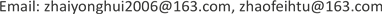1. 引言

2. 相关模型介绍2.1. VaR

VaR称为在险价值是由摩根公司首先提出，它显示了金融头寸在一定的持有期，以一定的概率所发生的最大损失  。其本质是收益率分布的分位数，因此定义一个多头头寸在持有期为t，置信度为p的

VaR为 VaR p = inf { x | F ( x ) ≥ p } ，其中inf表示满足 F ( x ) ≥ p 的最小实数。

L R = − 2 ln [ ( 1 − p ) T − N p N ] + 2 ln { [ 1 − ( N / T ) T − N ( N / T ) T ] }

2.2. GARCH模型

1982年恩格尔在研究英国通货膨胀问题时发现时间序列在某些阶段波动持续偏大，在某些阶段又持续小幅波动，这种特征在统计学称为异方差性。为了更好的捕捉这些特征，恩格尔提出了ARCH模型对具有异方差特征的时间序列进行建模。而后波勒斯列夫又在ARCH模型的基础上提出了广义自回归条件异方差模型，以解决ARCH模型在高阶时的参数估计问题。一般GARCH模型可以表示为：

r t = φ o + ∑ i = 1 p φ i r t − i + a t − ∑ i = 1 q θ i a t − i

a t = σ t ε t σ t 2 = ω 0 + ∑ i = 1 m α i a t − i 2 + ∑ j = 1 s β j σ t − j 2

2.3. 极值理论

F ( x ) = { 1 − exp [ − ( 1 + k x ) 1 / k ] 若 k ≠ 0 1 − exp [ − exp ( x ) ] 若 k = 0

GEV的意义在于不管数据原始分布是什么样子的，其极值的渐进分布都服从广义极值分布。GEV标

F ( x ) = { 1 − exp [ − ( 1 + k x ) 1 / k ] , 若 x < − 1 / k 1 , 其 他

F ( x ) = { 1 − exp [ − ( 1 + k ) 1 / k ] , 若 x > − 1 / k 0 , 其 他

3. 模型建立及分析3.1. 数据选取

2009年比特币刚刚提出，市场化程度不高，还不为人所熟知，收益率数据不具有代表性。因此本文选取2012年1月1日至2016年12月30日以美元标价比特币的简单收益率为样本数据的时间序列图，如图1所示，可以看出比特币的简单收益率序列大部分时段呈现平稳态势，但在某些阶段波动持续偏大，某些阶段波动持续偏小。根据时序图初步判断简单收益率序列平稳，波动具有集聚性(数据来源https://cn.investing.com，数据处理采用R3.4.2)。

3.2. 描述性分析3.2.1. 正态性

3.2.2. 平稳性

3.2.3. 自相关性

3.3. 实证分析

3.3.1. GARCH模型拟合

Red pool information criteri

5.05185.05295.05285.0529

R t = μ t + ε t ; ε t = σ t e t ; σ t 2 = ω + α ( L ) ε t 2 + β ( L ) σ t 2

Parameter estimation result

μ 00.155640.0419453.71060.000207
μ 10.411170.2875283.48020.000501
θ 1−0.435550.283256−3.70840.000209
ω0.402370.0274603.48390.000494
α0.209420.0255727.62660.000000
β0.789580.17127630.87600.000000
k3.081850.17127817.99330.000000

Residual variance tes

Lag0.062280.8029
Lag0.460620.9636
Lag0.821230.9925

3.3.2. GEV模型拟合

Parameter estimation of generalized extremum distributio
GEV形态参数k位置参数µ尺度参数σ

3.3.3. VaR的计算与回测检验

Backtest results when the backtest sample size is 170

95%置信度预期失败天数8585

LR统计量10.737 (3.841)0.77 (3.841)
99%置信度预期失败天数1717

LR统计量24.533 (6.635)0.061 (6.635)

Results of backtest when the sample size of backtest is 150

95%置信度预期失败天数7575

LR统计量0.221 (3.841)0.125 (3.841)
99%置信度预期失败天数1515

LR统计量0.258 (6.635)0.282 (6.635)

4. 结论